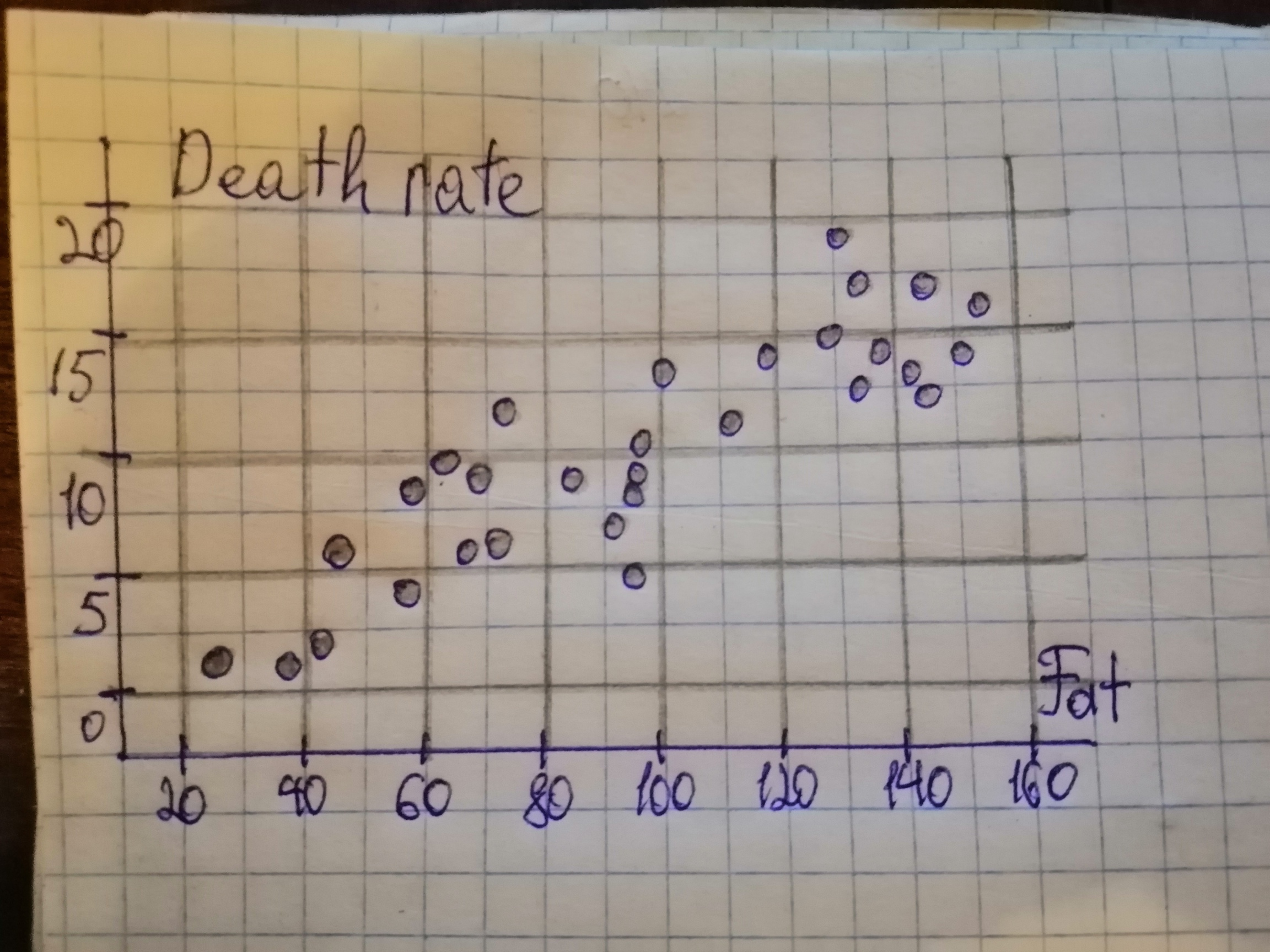Question# Researchers have asked whether there is a relationship between nutrition and cancer, and many studies have shown that there is. In fact, one of the co

Scatterplots
ANSWEREDResearchers have asked whether there is a relationship between nutrition and cancer, and many studies have shown that there is. In fact, one of the conclusions of a study by B. Reddy et al., “Nutrition and Its Relationship to Cancer” (Advances in Cancer Research, Vol. 32, pp. 237-345), was that “...none of the risk factors for cancer is probably more significant than diet and nutrition.” One dietary factor that has been studied for its relationship with prostate cancer is fat consumption. On the WeissStats CD, you will find data on per capita fat consumption (in grams per day) and prostate cancer death rate (per 100,000 males) for nations of the world. The data were obtained from a graph-adapted from information in the article mentioned-in J. Robbins’s classic book Diet for a New America (Walpole, NH: Stillpoint, 1987, p. 271). For part (d), predict the prostate cancer death rate for a nation with a per capita fat consumption of 92 grams per day. a) Construct and interpret a scatterplot for the data. b) Decide whether finding a regression line for the data is reasonable. If so, then also do parts (c)-(f). c) Determine and interpret the regression equation. d) Make the indicated predictions. e) Compute and interpret the correlation coefficient. f) Identify potential outliers and influential observations.2021-02-26

Given: $$\displaystyle{n}=\ \text{Sample size}\ ={30}$$ a) Fat is on the horizontal axis and Death rate is on the vertical axis.b) When there is no strong curvature presents in the scatterplot, then it is safe to assume that there is a linear relationship between the variables and thus it is then reasonable to find a regression line. We note that the scatterplot of part (a) does not contain strong curvature and thus it is reasonable to find the regression line. c) We determine all necessary sums: $$\displaystyle\sum\ {x}_{{{i}}}={2911}$$
$$\displaystyle\sum\ {y}_{{{i}}}={298.1}$$
$$\displaystyle\sum\ {x}_{{{i}}}\ {y}_{{{i}}}={33630.1}$$
$$\displaystyle\sum\ {{x}_{{{i}}}^{{{2}}}}={323963}$$
$$\displaystyle\sum\ {{y}_{{{i}}}^{{{2}}}}={3642.85}$$ Next, we can determine $$\displaystyle{S}_{{ x x}}\ \text{and}\ {S}_{{{x}{y}}}$$
$$\displaystyle{S}_{{xx}}=\ \sum\ {{x}_{{{i}}}^{{{2}}}}\ -\ {\frac{{{\left(\sum\ {x}_{{{i}}}\right)}^{{{2}}}}}{{{n}}}}={323963}\ -\ {\frac{{{2911}^{{{2}}}}}{{{30}}}}={41498.9667}$$
$$\displaystyle{S}_{{{x}{y}}}=\ \sum\ {x}_{{{i}}}\ {y}_{{{i}}}\ -\ {\frac{{{\left(\sum\ {x}_{{{i}}}\right)}{\left(\sum\ {y}_{{{i}}}\right)}}}{{{n}}}}={33630.1}\ -\ {\frac{{{2911}\ \cdot\ {298.1}}}{{{30}}}}={4704.4633}$$ The estimate b of the slope $$\displaystyle\beta\ \text{is the ratio of}\ {S}_{{{x}{y}}}\ \text{and}\ {S}_{{x x}}:$$
$$\displaystyle{b}=\ {\frac{{{S}_{{{x}{y}}}}}{{{S}_{{x x}}}}}=\ {\frac{{{4704.4633}}}{{{41498.9667}}}}={0.1134}$$ The mean is the sum of all values divided by the number of values: $$\displaystyle\overline{{{x}}}=\ {\frac{{\sum\ {x}_{{{i}}}}}{{{n}}}}=\ {\frac{{{2911}}}{{{30}}}}={97.0333}$$
$$\displaystyle\overline{{{y}}}=\ {\frac{{\sum\ {y}_{{{i}}}}}{{{n}}}}=\ {\frac{{{298.1}}}{{{30}}}}={9.9367}$$ The estimate a of the intercept $$\displaystyle\alpha$$ is the average of y decreased by the product of the estimate of the slope and the average of x. $$\displaystyle{a}=\ \overline{{{y}}}\ -\ {b}\ \overline{{{x}}}={9.9367}\ -\ {0.1134}\ \cdot\ {97.0333}=\ -{1.0634}$$ Generel least-squares equation: $$\displaystyle\hat{{{y}}}=\ \alpha\ +\ \beta\ {x}.\ \text{Replace}\ \alpha\ \text{by}\ {a}=\ -{1.0634}\ \text{and}\ \beta\ \text{by}\ {b}={0.1134}\ \text{in the general least-squares equation:}$$
$$\displaystyle\hat{{{y}}}={a}\ +\ {b}{x}=\ -{1.0634}\ +\ {0.1134}{x}$$ d) Let us evalute the regression line in part (c) at $$\displaystyle{x}={92}:$$
$$\displaystyle\hat{{{y}}}=\ -{1.0634}\ +\ {0.1134}{\left({92}\right)}={9.3661}$$ Thus the predicted death rate for a nation with a capita fat consumption of 92 grams per day is 9.3661 deaths per 100,000 males. e) We determine all necessary sums: $$\displaystyle\sum\ {x}_{{{i}}}={2911}$$
$$\displaystyle\sum\ {y}_{{{i}}}={298.1}$$
$$\displaystyle\sum\ {x}_{{{i}}}\ {y}_{{{i}}}={33630.1}$$
$$\displaystyle\sum\ {{x}_{{{i}}}^{{{2}}}}={323963}$$
$$\displaystyle\sum\ {{y}_{{{i}}}^{{{2}}}}={3642.85}$$ Determine the correlation coefficient: $$\displaystyle{r}=\ {\frac{{\sum\ {x}_{{{i}}}\ {y}_{{{i}}}\ -\ {\left(\sum\ {x}_{{{i}}}\right)}\frac{{\sum\ {y}_{{{i}}}}}{{n}}}}{{\sqrt{{{\left[\sum\ {{x}_{{{i}}}^{{{2}}}}\ -\ \frac{{\left(\sum\ {x}_{{{i}}}\right)}^{{{2}}}}{{n}}\right]}{\left[\sum\ {{y}_{{{i}}}^{{{2}}}}\ -\ \frac{{\left(\sum\ {y}_{{{i}}}\right)}^{{{2}}}}{{n}}\right]}}}}}}$$
$$\displaystyle=\ {\frac{{{3363.1}-\ {\left({2911}\right)}\frac{{{298.1}}}{{30}}}}{{\sqrt{{{\left[{323963}\ -\ \frac{{2911}^{{{2}}}}{{30}}\right]}{\left[{3642.85}\ -\ \frac{{298.1}^{{{2}}}}{{30}}\right]}}}}}}$$
$$\displaystyle\approx{0.8851}$$ If r is positive, then there is a positive linear relationship. If r is negative, then there is a negative linear relationship. If $$\displaystyle{0}\ {<}\ {\left|{r}\right|}\ {<}\ {0.5},\ \text{then the linear relationship is weak. If}\ {0.5}\ {<}\ {\left|{r}\right|}\ {<}\ {0.8},\ \text{then the linear relationship is moderate. If}\ {0.8}\ {<}\ {\left|{r}\right|}\ {<}\ {1},$$ then the linear relationship is strong. We note that the linear correlation coefficient r is greater than 0.8 in absolute value and positive, thus there is a strong positive linear relationship between the variables. f) There are no points in the scatterplot that deviate strongly from the general pattern in the scatterplot of part (a) and thus there appear to be no outliers. Since there are no outliers, there are no influential observations either.